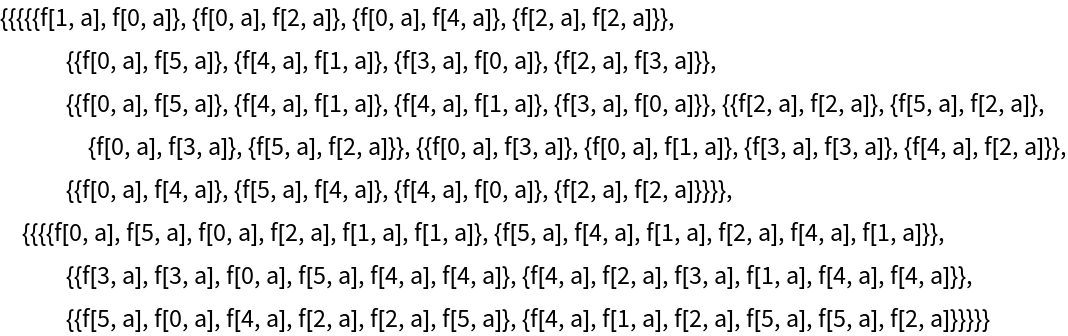Function Repository Resource:

A version of MapThread that allows for ragged arrays and for lists with unequal depth

Contributed by: Sander Huisman
 ResourceFunction["GeneralizedMapThread"][f,{{a1,a2,…},{b1,b2,…},…}] gives {f[a1,b1,…],f[a2,b2,…],…}. ResourceFunction["GeneralizedMapThread"][f,{expr1,expr2,…},n] applies f to the parts of the expri at level n. ResourceFunction["GeneralizedMapThread"][f] represents an operator form of ResourceFunction["GeneralizedMapThread"] that can be applied to an expression.

Details and Options

If the lists do not have equal length, the smallest lists are made equal length by making copies using ConstantArray.

Examples

Basic Examples

Have the second argument be a constant:

 In:=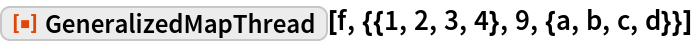Out=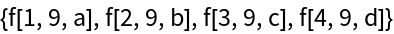It works on expressions with unequal lengths (“ragged”):

 In:=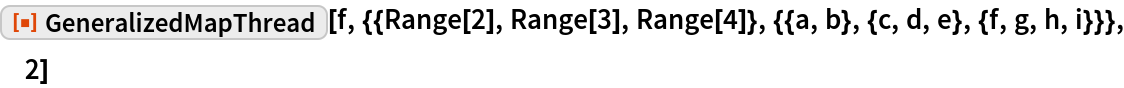Out=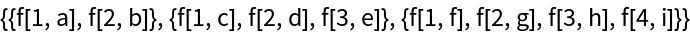Apply f to the corresponding values of associations, copying values where necessary:

 In:=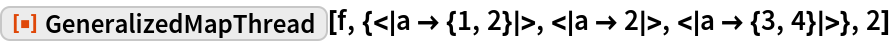Out=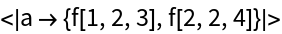Scope

Apply to a list of lists with varying depths and lengths:

 In:=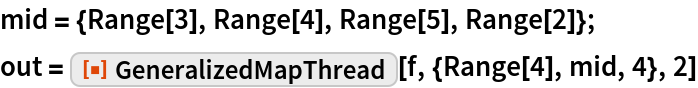Out=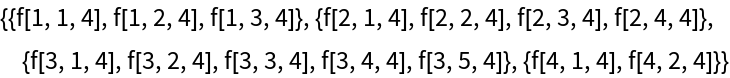The final results are ragged:

 In:=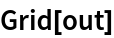Out=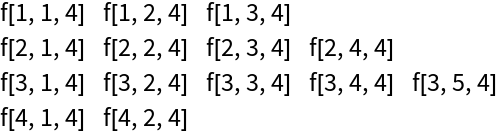Apply at level 1 means that {a,b} from the final list is considered a single value:

 In:=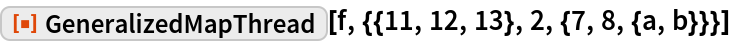Out=Apply at level 2 means interpreting {a,b} as separate values, and copying all the other values:

 In:=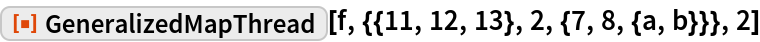Out=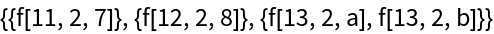In:=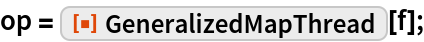Use the operator on some data:

 In:=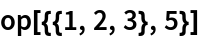Out=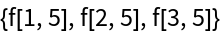Applications

Subtract the vector {a,b} from a list of vectors:

 In:=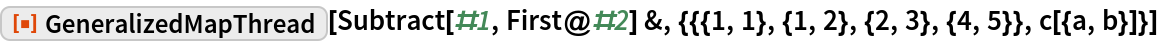Out=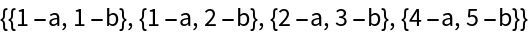Apply a list of functions to a single argument without pure functions:

 In:=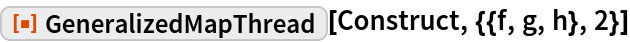Out=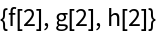This can also be achieved using Through:

 In:=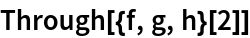Out=Properties and Relations

When the arrays are of equal length, MapThread and GeneralizedMapThread give the same result:

 In:=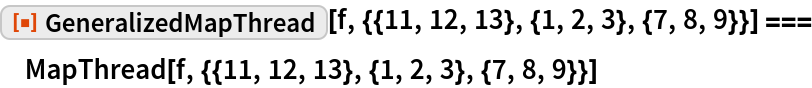Out=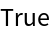MapThread cannot handle lists with elements of unequal length (“ragged”):

 In:=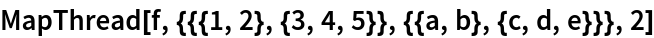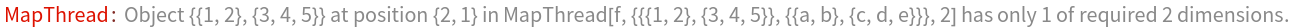Out=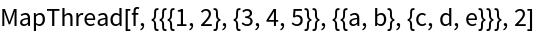In:=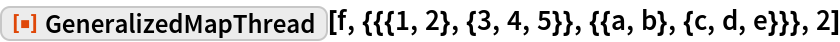Out=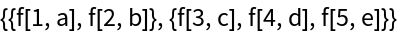Thread can handle arrays with different depths:

 In:=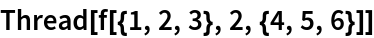Out=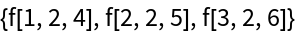In:=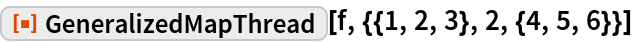Out=Possible Issues

Associations must have the same keys:

 In:=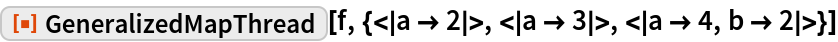Out=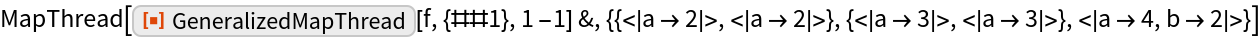Associations and lists cannot be mixed:

 In:=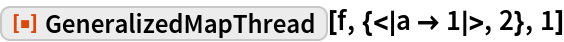Out=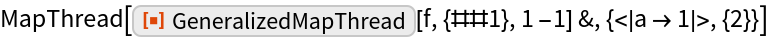Values with another head, such as List or Association, or an incorrect length will get copied:

 In:=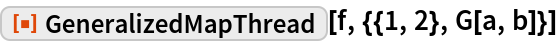Out=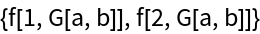For the case of unequal length, the longest list is taken as the actual length. Here the last list is the longest; the first list is copied:

 In:=Out=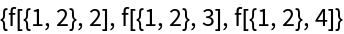Here the first list is the longest; the last list is copied:

 In:=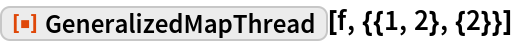Out=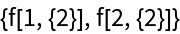An extra bracket around the second list will make it the shortest:

 In:=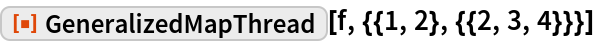Out=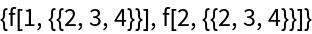One can also give it a different head, which prevents it from being interpreted as a list:

 In:=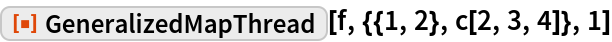Out=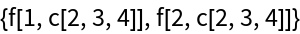Neat Examples

Interpret the entries as three levels deep, copying them all:

 In:=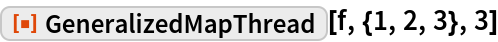Out=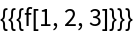Create a complex ragged array with different depths:

 In:=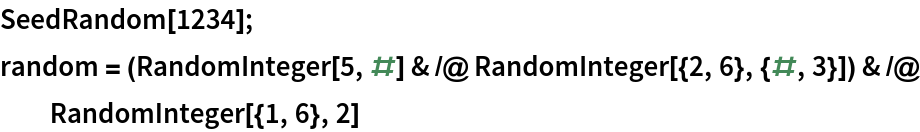Out=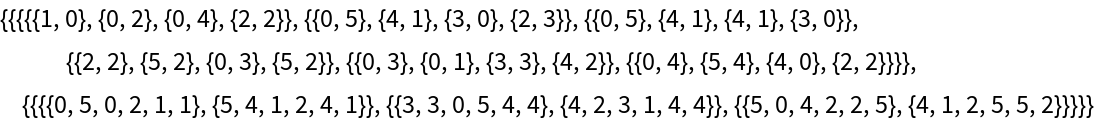Apply the function f five levels deep, with the second argument set constant to a:

 In:=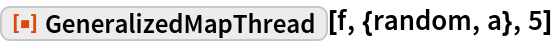Out=